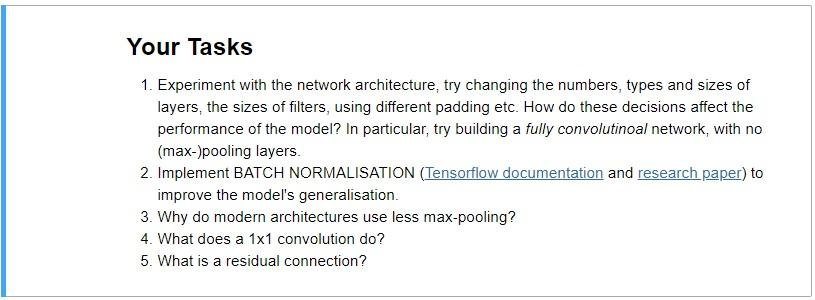# Python convolutional neural network exercise

USE Jupyter Notebook

We will combine what we’ve learned about convolution, max-pooling and feed-forward layers, to build a ConvNet classifier for images.

Given Code:

in[]from __future__ import absolute_import, division, print_function

# Prerequisits
!pip install pydot_ng
!pip install graphviz
!apt install graphviz > /dev/null

# import statements
import tensorflow as tf
import tensorflow.contrib.eager as tfe
import numpy as np
import matplotlib.pyplot as plt
from IPython import display
%matplotlib inline

# Enable the interactive TensorFlow interface, which is easier to understand as a beginner.
try:
tf.enable_eager_execution()
print(‘Running in Eager mode.’)
except ValueError:

in[]cifar = tf.keras.datasets.cifar10
(train_images, train_labels), (test_images, test_labels) = cifar.load_data()
cifar_labels = [‘airplane’, ‘automobile’, ‘bird’, ‘cat’, ‘deer’, ‘dog’, ‘frog’, ‘horse’, ‘ship’, ‘truck’]

in[]# Take the last 10000 images from the training set to form a validation set
train_labels = train_labels.squeeze()
validation_images = train_images[-10000:, :, :]
validation_labels = train_labels[-10000:]
train_images = train_images[:-10000, :, :]
train_labels = train_labels[:-10000]

in[]print(‘train_images.shape = {}, data-type = {}’.format(train_images.shape, train_images.dtype))
print(‘train_labels.shape = {}, data-type = {}’.format(train_labels.shape, train_labels.dtype))

print(‘validation_images.shape = {}, data-type = {}’.format(validation_images.shape, validation_images.dtype))
print(‘validation_labels.shape = {}, data-type = {}’.format(validation_labels.shape, validation_labels.dtype))

in[]plt.figure(figsize=(10,10))
for i in range(25):
plt.subplot(5,5,i+1)
plt.xticks([])
plt.yticks([])
plt.grid(‘off’)

in[]# Define the convolutinal part of the model architecture using Keras Layers.
model = tf.keras.models.Sequential([
tf.keras.layers.Conv2D(filters=48, kernel_size=(3, 3), activation=tf.nn.relu, input_shape=(32, 32, 3), padding=’same’),
tf.keras.layers.MaxPooling2D(pool_size=(3, 3)),
tf.keras.layers.MaxPooling2D(pool_size=(3, 3)),
tf.keras.layers.MaxPooling2D(pool_size=(3, 3)),

in[]model.summary()

in[]model.add(tf.keras.layers.Flatten()) # Flatten “squeezes” a 3-D volume down into a single vector.

in[]tf.keras.utils.plot_model(model, to_file=”small_lenet.png”, show_shapes=True, show_layer_names=True)
display.display(display.Image(‘small_lenet.png’))

in[]batch_size = 128
num_epochs = 10 # The number of epochs (full passes through the data) to train for

# Compiling the model adds a loss function, optimiser and metrics to track during training
loss=tf.keras.losses.sparse_categorical_crossentropy,
metrics=[‘accuracy’])

# The fit function allows you to fit the compiled model to some training data
model.fit(x=train_images,
y=train_labels,
batch_size=batch_size,
epochs=num_epochs,
validation_data=(validation_images, validation_labels.astype(np.float32)))

print(‘Training complete’)

in[]metric_values = model.evaluate(x=test_images, y=test_labels)

print(‘Final TEST performance’)
for metric_value, metric_name in zip(metric_values, model.metrics_names):
print(‘{}: {}’.format(metric_name, metric_value))

in[]img_indices = np.random.randint(0, len(test_images), size=)
sample_test_images = test_images[img_indices]
sample_test_labels = [cifar_labels[i] for i in test_labels[img_indices].squeeze()]

predictions = model.predict(sample_test_images)
max_prediction = np.argmax(predictions, axis=1)
prediction_probs = np.max(predictions, axis=1)

in[]plt.figure(figsize=(10,10))
for i, (img, prediction, prob, true_label) in enumerate(
zip(sample_test_images, max_prediction, prediction_probs, sample_test_labels)):
plt.subplot(5,5,i+1)
plt.xticks([])
plt.yticks([])
plt.grid(‘off’)

plt.imshow(img)
plt.xlabel(‘{} ({:0.3f})’.format(cifar_labels[prediction], prob))
plt.ylabel(‘{}’.format(true_label))

Tensorflow documentation: from ‘tensorflow.org’ website: search: tf.keras.layers.BatchNormalization’

research paper: search on web: ‘proceedings.mlr.press/v37/ioffe15.pdf’Pages (550 words)
Approximate price: -

Why Choose UsQuality Papers

We value our clients. For this reason, we ensure that each paper is written carefully as per the instructions provided by the client. Our editing team also checks all the papers to ensure that they have been completed as per the expectations.Over the years, our Acme Homework has managed to secure the most qualified, reliable and experienced team of writers. The company has also ensured continued training and development of the team members to ensure that it keep up with the rising Academic Trends.Affordable Prices

Our prices are fairly priced in such a way that ensures affordability. Additionally, you can get a free price quotation by clicking on the "Place Order" button.On-Time delivery

We pay strict attention on deadlines. For this reason, we ensure that all papers are submitted earlier, even before the deadline indicated by the customer. For this reason, the client can go through the work and review everything.100% Originality

At Essay USA, all papers are plagiarism-free as they are written from scratch. We have taken strict measures to ensure that there is no similarity on all papers and that citations are included as per the standards set.Our support team is readily available to provide any guidance/help on our platform at any time of the day/night. Feel free to contact us via the Chat window or support email: support@acmehomework.com.

Try it now!

## Calculate the price of your order

We'll send you the first draft for approval by at
Total price:
\$0.00

How it works?Fill in the order form and provide all details of your assignment.Proceed with the payment

Choose the payment system that suits you most.Our Services

Essay USA has stood as the world’s leading custom essay writing services providers. Once you enter all the details in the order form under the place order button, the rest is up to us.## Essay Writing Services

At Essay USA, we prioritize on all aspects that bring about a good grade such as impeccable grammar, proper structure, zero-plagiarism and conformance to guidelines. Our experienced team of writers will help you completed your essays and other assignments.Be assured that you’ll definitely get accepted to the Master’s level program at any university once you enter all the details in the order form. We won’t leave you here; we will also help you secure a good position in your aspired workplace by creating an outstanding resume or portfolio once you place an order.